# Classes and Data Abstraction Topic 5 Introduction Objectoriented

• Slides: 20
Download presentationClasses and Data Abstraction Topic 5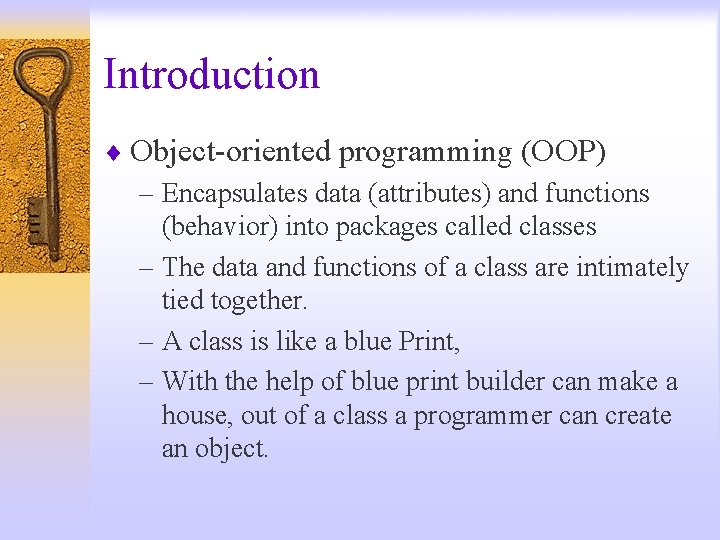Introduction ¨ Object-oriented programming (OOP) – Encapsulates data (attributes) and functions (behavior) into packages called classes – The data and functions of a class are intimately tied together. – A class is like a blue Print, – With the help of blue print builder can make a house, out of a class a programmer can create an object.Introduction – One blue print can be reused many times to make many houses, similarly one class can be reused many times to make many objects of the same class. – Procedural language programming tends to be action oriented, c++ programming is object oriented. – The unit of the programming in c is the function where in C++ is the class – Classes are also referred to as programmer defined types.Introduction – Each class contains data as well as the set of functions that manipulate the data. – The data components of a class are called data members. The function components of a class are called member function. ¨ Information hiding – Class objects communicate across well-defined interfaces – Implementation details hidden within classes themselves ¨ User-defined (programmer-defined) types: classes – Data (data members) – Functions (member functions or methods) – Similar to blueprints – reusable – Class instance: object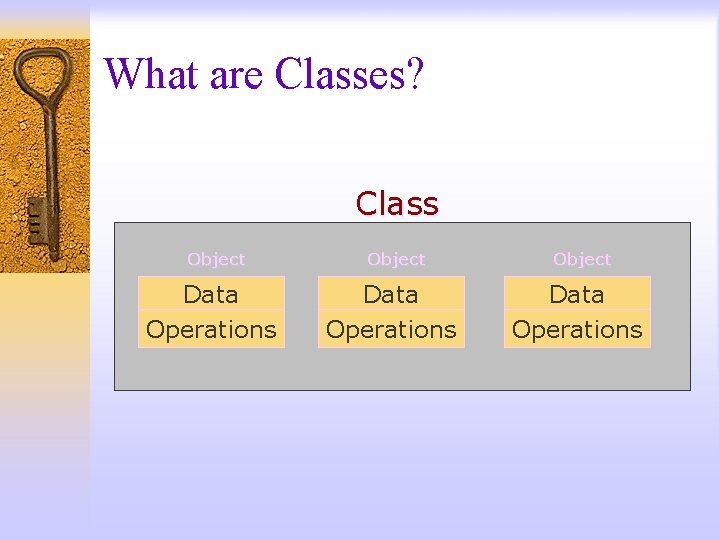What are Classes? Class Object Data Operations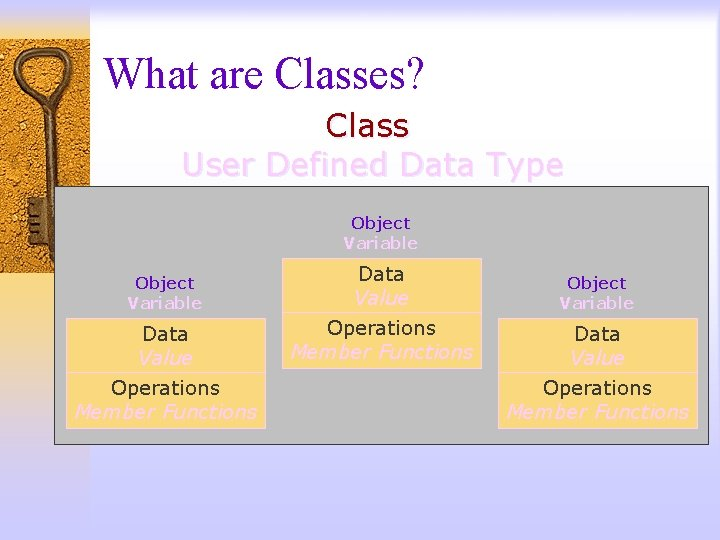What are Classes? Class User Defined Data Type Object Variable Data Value Operations Member FunctionsWhat are Classes? ¨ A class is a data type ¨ You can use classes in the same way you use predefined data types (int, char, etc. ) ¨ Defining your class the right way is important for it to behave like predefined data types Abstract Data Type (ADT) ¨ An ADT is a user-defined data type that is well behaved as the predefined data typesStructures ¨ A data structure that can be used to store related data items with different types. ¨ The individual components of a struct is called a member.Structures Students ID Name Major 1111 Nora CS 2222 Sara IS 3333 Mona CS n n n Student: ID variable Student: Name variable Student: Major variable ¨ Student – ID – Name – MajorStructures Think of a structure as an object without any member functions Object Variable Data Value Operations Member Functions Here, we’ll have values of different data types that we would like to treat as a single item.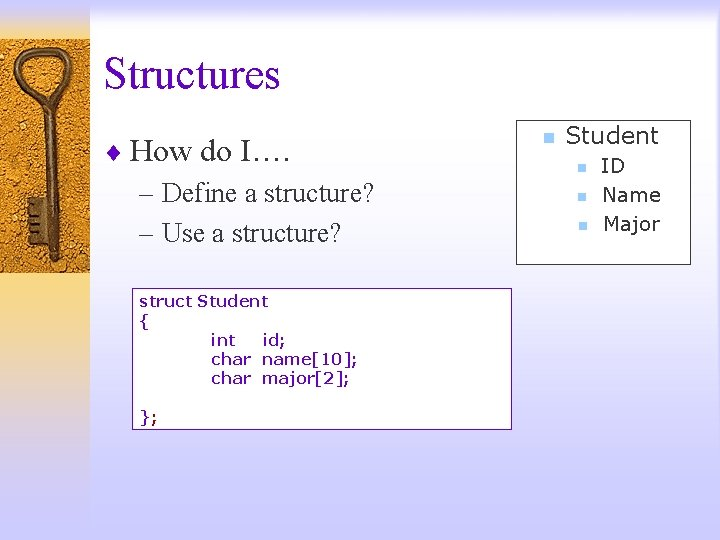Structures ¨ How do I…. – Define a structure? – Use a structure? struct Student { int id; char name; char major; }; n Student n n n ID Name Major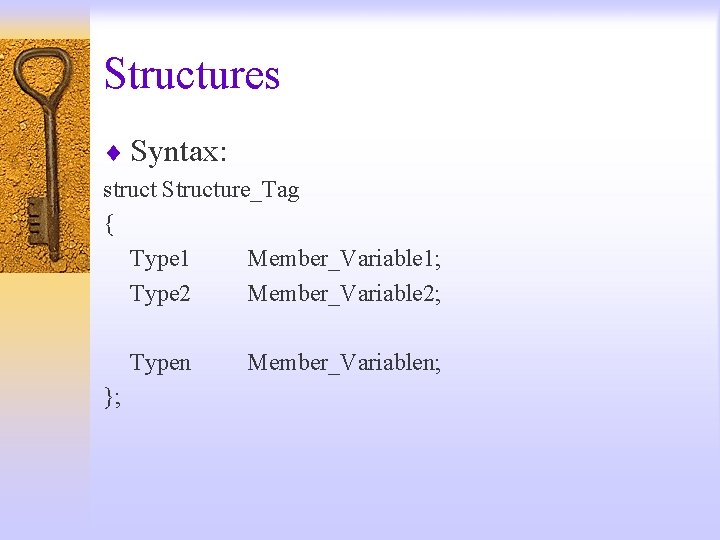Structures ¨ Syntax: struct Structure_Tag { Type 1 Member_Variable 1; Type 2 Member_Variable 2; Typen }; Member_Variablen;Structures ¨ Using Structures – Declare: Student 1, Student 2; – Assignment: Student 1 = Student 2; Student. Record • Student 1. id = Student 2. id; • Student 1. grade = Student 2. grade; – Read: cin >> Student 1. id; – Write: cout << Student 1. id; – Initialize: Student 1 = {666, ’A’}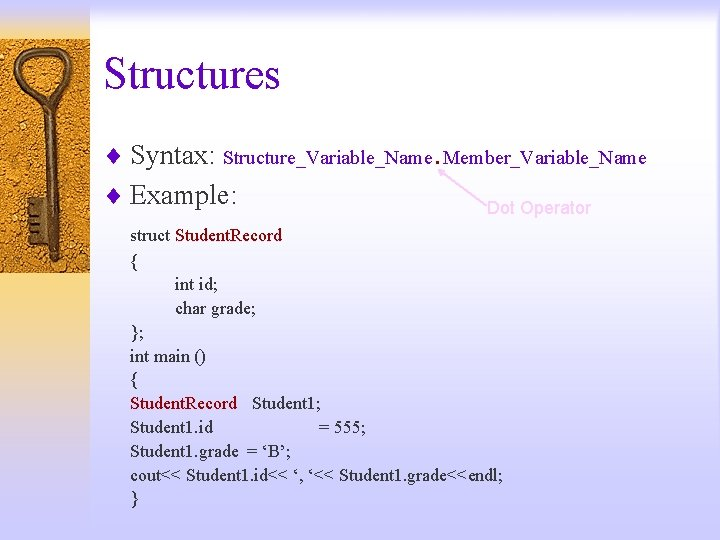Structures ¨ Syntax: Structure_Variable_Name. Member_Variable_Name ¨ Example: Dot Operator struct Student. Record { int id; char grade; }; int main () { Student. Record Student 1; Student 1. id = 555; Student 1. grade = ‘B’; cout<< Student 1. id<< ‘, ‘<< Student 1. grade<<endl; }Structures ¨ Two or more structure types may use the same member names struct Fertilizer. Stock { double quantity; double nitrogen_content; }; struct Crop. Yield { int quantity; double size; }; Fertilizer. Stock Item 1; Item 1. quantity Crop. Yield Apples; Apples. quantityStructures ¨ Structures within structures (nested) struct Date { int month; int day; int year; }; struct Employee { int id; Date birthday; }; Employee person 1; cout<< person 1. birthday. year;Structures #include <iostream> struct Student. Record { int id; char grade; }; Student. Record Get_Data (Student. Record in_student); int main () { using namespace std; Student. Record Student 1; Student 1 = Get_Data (Student 1); cout<< Student 1. id<< ", "<<Student 1. grade<< endl; return 0; } Student. Record Get_Data (Student. Record in_student) { using namespace std; cout<<"Enter ID: "; cin>> in_student. id; cout<<"Enter Grade: "; cin>> in_student. grade; return (in_student); }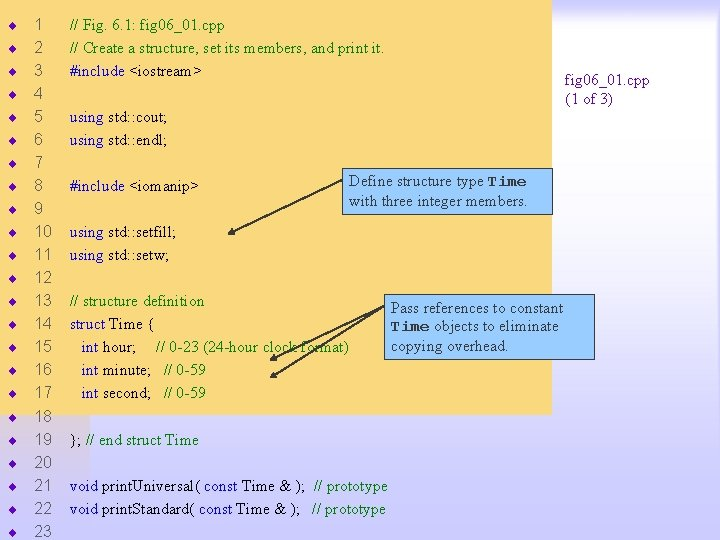¨ ¨ ¨ ¨ ¨ ¨ 1 2 3 4 5 6 7 8 9 10 11 12 13 14 15 16 17 18 19 20 21 22 23 // Fig. 6. 1: fig 06_01. cpp // Create a structure, set its members, and print it. #include <iostream> fig 06_01. cpp (1 of 3) using std: : cout; using std: : endl; #include <iomanip> Define structure type Time with three integer members. using std: : setfill; using std: : setw; // structure definition struct Time { int hour; // 0 -23 (24 -hour clock format) int minute; // 0 -59 int second; // 0 -59 }; // end struct Time void print. Universal( const Time & ); // prototype void print. Standard( const Time & ); // prototype Pass references to constant Time objects to eliminate copying overhead.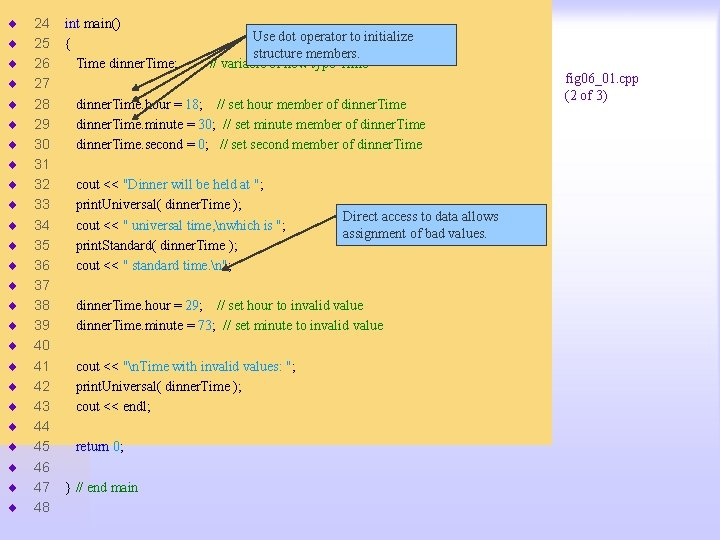¨ ¨ ¨ ¨ ¨ ¨ ¨ 24 25 26 27 28 29 30 31 32 33 34 35 36 37 38 39 40 41 42 43 44 45 46 47 48 int main() { Time dinner. Time; Use dot operator to initialize structure members. // variable of new type Time dinner. Time. hour = 18; // set hour member of dinner. Time. minute = 30; // set minute member of dinner. Time. second = 0; // set second member of dinner. Time cout << "Dinner will be held at "; print. Universal( dinner. Time ); cout << " universal time, nwhich is "; print. Standard( dinner. Time ); cout << " standard time. n"; Direct access to data allows assignment of bad values. dinner. Time. hour = 29; // set hour to invalid value dinner. Time. minute = 73; // set minute to invalid value cout << "n. Time with invalid values: "; print. Universal( dinner. Time ); cout << endl; return 0; } // end main fig 06_01. cpp (2 of 3)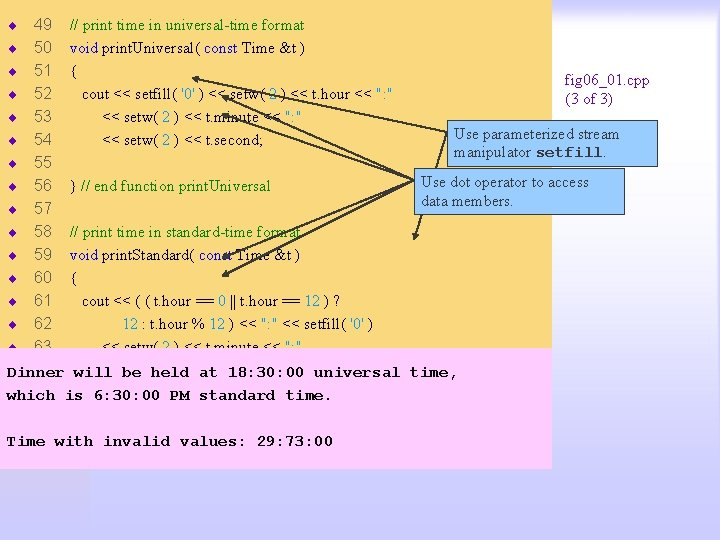¨ 49 // print time in universal-time format ¨ 50 void print. Universal( const Time &t ) ¨ 51 { fig 06_01. cpp ¨ 52 cout << setfill( '0' ) << setw( 2 ) << t. hour << ": " (3 of 3) ¨ 53 << setw( 2 ) << t. minute << ": " Use parameterized stream fig 06_01. cpp ¨ 54 << setw( 2 ) << t. second; manipulator setfill. output (1 of 1) ¨ 55 Use dot operator to access ¨ 56 } // end function print. Universal data members. ¨ 57 ¨ 58 // print time in standard-time format ¨ 59 void print. Standard( const Time &t ) ¨ 60 { ¨ 61 cout << ( ( t. hour == 0 || t. hour == 12 ) ? ¨ 62 12 : t. hour % 12 ) << ": " << setfill( '0' ) ¨ 63 << setw( 2 ) << t. minute << ": " ¨ 64 will << be setw(held 2 ) <<at t. second Dinner 18: 30: 00 universal time, ¨ 65 is 6: 30: 00 << ( t. hour. PM < 12 standard ? " AM" : " PM" ); which time. ¨ 66 ¨ 67 with } // end function print. Standard Time invalid values: 29: 73: 00